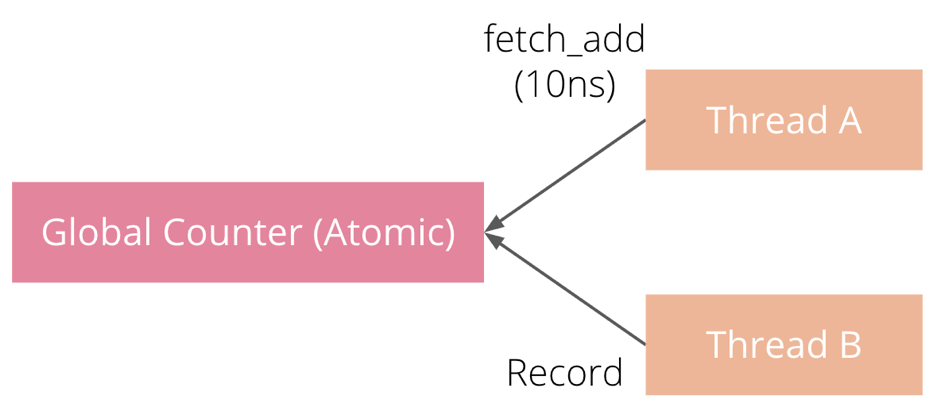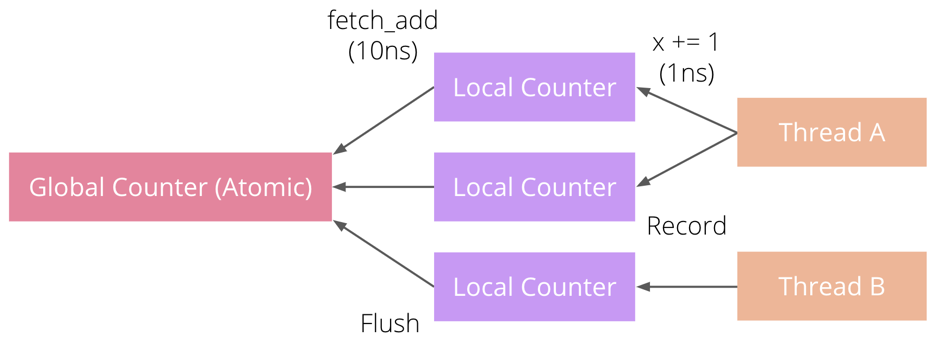2019-03-13

## 指标向量（Metric Vector）

Metric Vector 用于支持带 Label 的指标。由于各种指标都可以带上 Label，因此 Metric Vector 本身实现为了一种泛型结构体，`Counter``Gauge``Histogram` 在这之上实现了 `CounterVec``GaugeVec``HistogramVec`。Metric Vector 主要实现位于 src/vec.rs

``````pub type HistogramVec = MetricVec<HistogramVecBuilder>;
pub struct MetricVec<T: MetricVecBuilder> {
pub(crate) v: Arc<MetricVecCore<T>>,
}
impl<T: MetricVecBuilder> MetricVec<T> {
pub fn with_label_values(&self, vals: &[&str]) -> T::M {
self.get_metric_with_label_values(vals).unwrap()
}
}``````

``````pub trait MetricVecBuilder: Send + Sync + Clone {
type M: Metric;
type P: Describer + Sync + Send + Clone;
fn build(&self, &Self::P, &[&str]) -> Result<Self::M>;
}
pub(crate) struct MetricVecCore<T: MetricVecBuilder> {
pub children: RwLock<HashMap<u64, T::M>>,
// Some fields are omitted.
}
impl<T: MetricVecBuilder> MetricVecCore<T> {
// Some functions are omitted.
pub fn get_metric_with_label_values(&self, vals: &[&str]) -> Result<T::M> {
let h = self.hash_label_values(vals)?;
if let Some(metric) = self.children.read().get(&h).cloned() {
return Ok(metric);
}
self.get_or_create_metric(h, vals)
}
pub(crate) fn hash_label_values(&self, vals: &[&str]) -> Result<u64> {
if vals.len() != self.desc.variable_labels.len() {
return Err(Error::InconsistentCardinality(
self.desc.variable_labels.len(),
vals.len(),
));
}
let mut h = FnvHasher::default();
for val in vals {
h.write(val.as_bytes());
}
Ok(h.finish())
}
fn get_or_create_metric(&self, hash: u64, label_values: &[&str]) -> Result<T::M> {
let mut children = self.children.write();
// Check exist first.
if let Some(metric) = children.get(&hash).cloned() {
return Ok(metric);
}
let metric = self.new_metric.build(&self.opts, label_values)?;
children.insert(hash, metric.clone());
Ok(metric)
}
}``````

## 整型指标（Integer Metric）

`Atomic` trait 定义如下（src/atomic64/mod.rs）：

``````pub trait Atomic: Send + Sync {
/// The numeric type associated with this atomic.
type T: Number;
/// Create a new atomic value.
fn new(val: Self::T) -> Self;
/// Set the value to the provided value.
fn set(&self, val: Self::T);
/// Get the value.
fn get(&self) -> Self::T;
/// Increment the value by a given amount.
fn inc_by(&self, delta: Self::T);
/// Decrement the value by a given amount.
fn dec_by(&self, delta: Self::T);
}``````

``````pub struct Value<P: Atomic> {
pub val: P,
// Some fields are omitted.
}
pub struct GenericCounter<P: Atomic> {
v: Arc<Value<P>>,
}
pub type Counter = GenericCounter<AtomicF64>;
pub type IntCounter = GenericCounter<AtomicI64>;``````

## 本地指标（Local Metrics）

rust-prometheus 中 Counter 和 Histogram 指标支持 `local()` 函数，该函数会返回一个该指标的本地实例。本地实例是一个非线程安全的实例，不能多个线程共享。例如，`Histogram::local()` 会返回 `LocalHistogram`。由于 Local Metrics 使用是本地变量，开销极小，因此可以放心地频繁更新 Local Metrics。用户只需定期调用 Local Metrics 的 `flush()` 函数将其数据定期同步到全局指标即可。一般来说 Prometheus 收集数据的间隔是 15s 到 1 分钟左右（由用户自行配置），因此即使是以 1s 为间隔进行 `flush()` 精度也足够了。TiKV 中大量运用了本地指标提升性能。例如，TiKV 的线程池一般都提供 `Context` 变量，`Context` 中存储了本地指标。线程池上运行的任务都能访问到一个和当前 worker thread 绑定的 `Context`，因此它们都可以安全地更新 `Context` 中的这些本地指标。最后，线程池一般提供 `tick()` 函数，允许以一定间隔触发任务，`tick()` 中 TiKV 会对这些 `Context` 中的本地指标进行 `flush()`

### Local Counter

`Counter` 的本地指标 `LocalCounter` 实现很简单，它是一个包含了计数器的结构体，该结构体提供了与 `Counter` 一致的接口方便用户使用。该结构体额外提供了 `flush()`，将保存的计数器的值作为增量值更新到全局指标：

``````pub struct GenericLocalCounter<P: Atomic> {
counter: GenericCounter<P>,
val: P::T,
}
pub type LocalCounter = GenericLocalCounter<AtomicF64>;
pub type LocalIntCounter = GenericLocalCounter<AtomicI64>;
impl<P: Atomic> GenericLocalCounter<P> {
// Some functions are omitted.
pub fn flush(&mut self) {
if self.val == P::T::from_i64(0) {
return;
}
self.counter.inc_by(self.val);
self.val = P::T::from_i64(0);
}
}``````

### Local Histogram

``````pub struct LocalHistogramCore {
histogram: Histogram,
counts: Vec<u64>,
count: u64,
sum: f64,
}
impl LocalHistogramCore {
// Some functions are omitted.
pub fn observe(&mut self, v: f64) {
// Try find the bucket.
let mut iter = self
.histogram
.core
.upper_bounds
.iter()
.enumerate()
.filter(|&(_, f)| v <= *f);
if let Some((i, _)) = iter.next() {
self.counts[i] += 1;
}
self.count += 1;
self.sum += v;
}
pub fn flush(&mut self) {
// No cached metric, return.
if self.count == 0 {
return;
}
{
let h = &self.histogram;
for (i, v) in self.counts.iter().enumerate() {
if *v > 0 {
h.core.counts[i].inc_by(*v);
}
}
h.core.count.inc_by(self.count);
h.core.sum.inc_by(self.sum);
}
self.clear();
}
}``````

## 静态指标（Static Metrics）

``````struct Metrics {
kv_get: Histogram,
kv_scan: Histogram,
kv_prewrite: Histogram,
kv_commit: Histogram,
// ...
}
impl Metrics {
fn new() -> Metrics {
Metrics {
kv_get: GRPC_MSG_HISTOGRAM_VEC.with_label_values(&["kv_get"]),
kv_scan: GRPC_MSG_HISTOGRAM_VEC.with_label_values(&["kv_scan"]),
kv_prewrite: GRPC_MSG_HISTOGRAM_VEC.with_label_values(&["kv_prewrite"]),
kv_commit: GRPC_MSG_HISTOGRAM_VEC.with_label_values(&["kv_commit"]),
// ...
}
}
}``````

``````@@ -102,10 +155,8 @@ fn make_callback<T: Debug + Send + 'static>() -> (Box<FnBox(T) + Send>, oneshot:
impl<T: RaftStoreRouter + 'static> tikvpb_grpc::Tikv for Service<T> {
fn kv_get(&self, ctx: RpcContext, mut req: GetRequest, sink: UnarySink<GetResponse>) {
-        let label = "kv_get";
-        let timer = GRPC_MSG_HISTOGRAM_VEC
-            .with_label_values(&[label])
-            .start_coarse_timer();
+        const LABEL: &str = "kv_get";
+        let timer = self.metrics.kv_get.start_coarse_timer();
let (cb, future) = make_callback();
let res = self.storage.async_get(``````

1. 代码繁琐，有大量重复的、或满足某些 pattern 的代码；
2. 如果还有另一个 Label 维度，那么需要维护的字段数量就会急剧膨胀（因为每一种值的组合都需要分配一个字段）。

``````make_static_metric! {
pub struct GrpcMsgHistogram: Histogram {
"type" => {
kv_get,
kv_scan,
kv_prewrite,
kv_commit,
// ...
},
}
}
let metrics = GrpcMsgHistogram::from(GRPC_MSG_HISTOGRAM_VEC);
// Usage:
metrics.kv_get.start_coarse_timer();``````